# 1. Locate the centroid of the shaded area. y = a cos TX L ㅏ늘 L...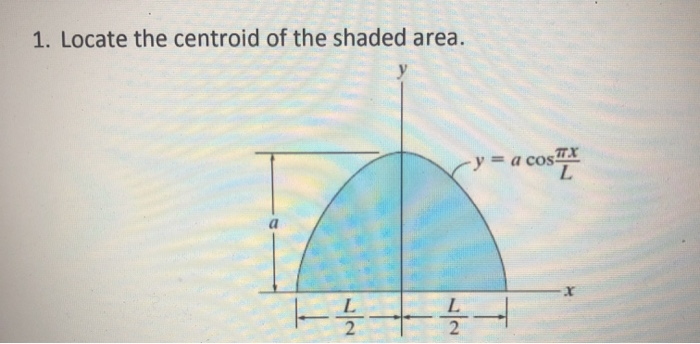1. Locate the centroid of the shaded area. y = a cos TX L ㅏ늘 L 2 늘 →

solution: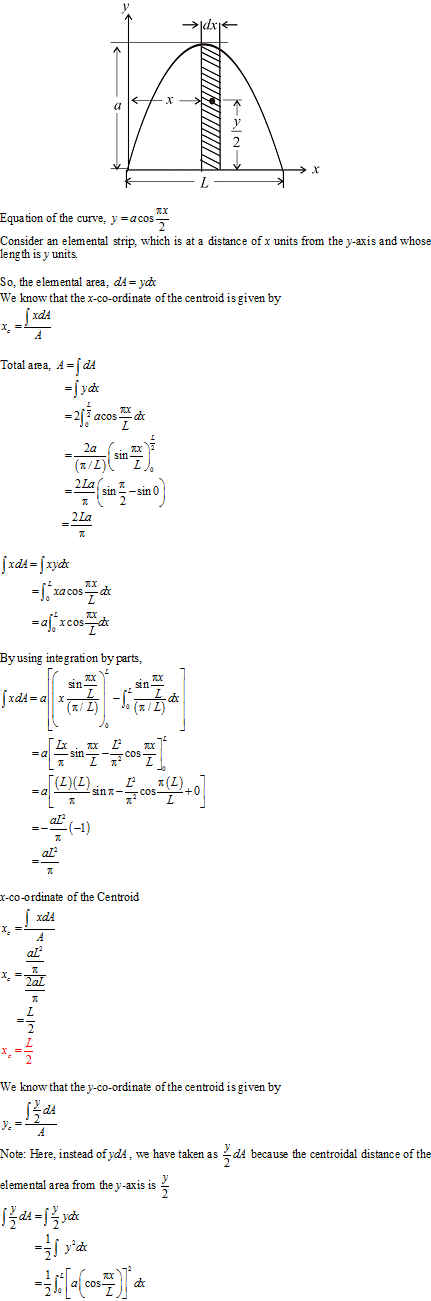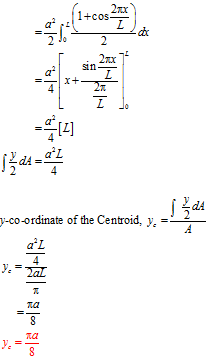##### Add Answer of: 1. Locate the centroid of the shaded area. y = a cos TX L ㅏ늘 L...
Similar Homework Help Questions
• ### Locate the centroid X of the shaded area, then locate centroid Y of the shaded area.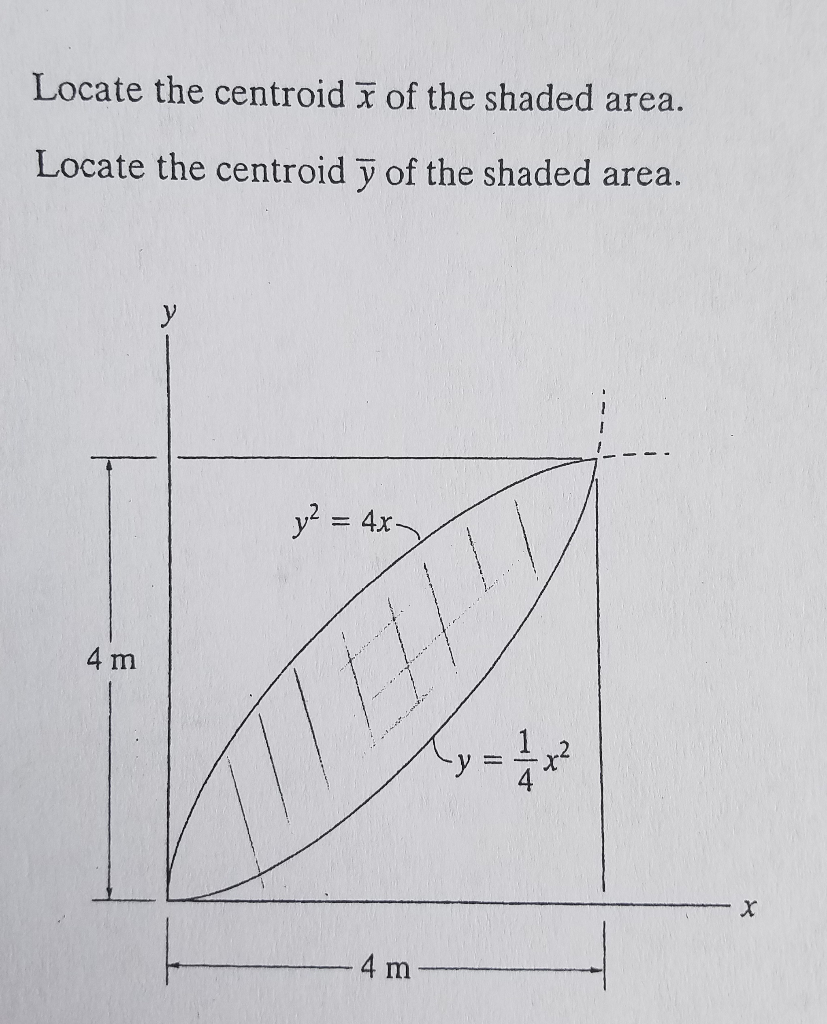Locate the centroid X of the shaded area, then locate centroid Y of the shaded area. Locate the centroid x of the shaded area. Locate the centroid y of the shaded area. 4 m 4 Locate the centroid x of the shaded area. Locate the centroid y of the shaded area. 4 m 4

• ### Locate the centroid of the shaded area between the two curves. Locate the centroid of the...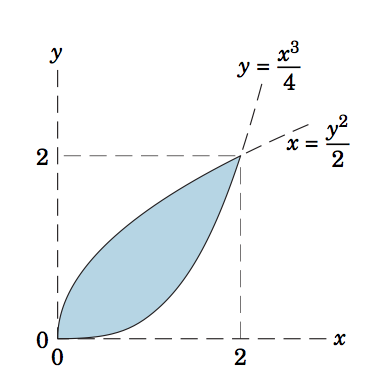Locate the centroid of the shaded area between the two curves. Locate the centroid of the shaded area between the two curves.

• ### 2. Locate the centroid y of the shaded area. у - an h x a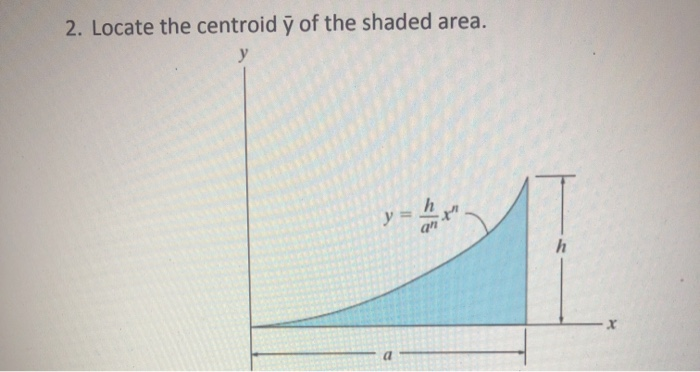2. Locate the centroid y of the shaded area. у - an h x a

• ### Locate the centroid (x, y) of the shaded area. Lifesaver!Locate the centroid (x, y) of the shaded area. Then find Ix and Iy.Lifesaver given to correct answer with all work shown.

• ### 3. Locate the centroid x of the shaded area. y = a sin a (TT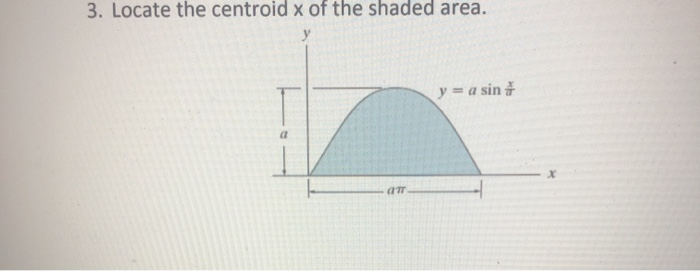3. Locate the centroid x of the shaded area. y = a sin a (TT

• ### 2) Locate the x coordinate of the centroid of the shaded area. 12" 3 6" 4...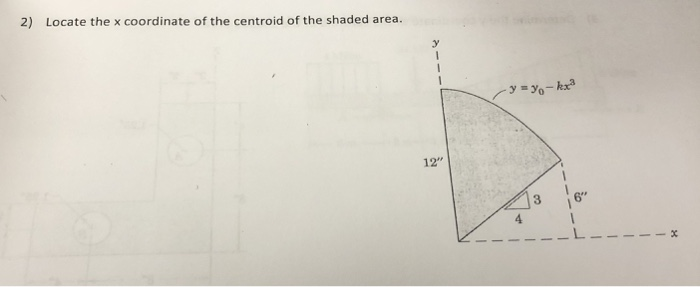2) Locate the x coordinate of the centroid of the shaded area. 12" 3 6" 4 ーし

• ### 9-15. Locate the centroid of the shaded area. Solve the problem by evaluating the integrals using...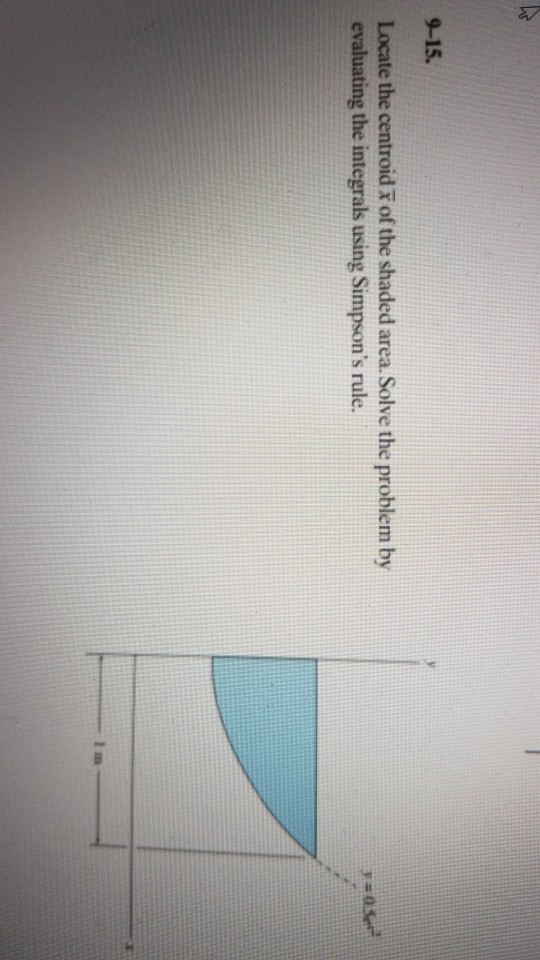9-15. Locate the centroid of the shaded area. Solve the problem by evaluating the integrals using Simpson's rule. =0

• ### Exercise 6.1.15 Calculate the area A of the shaded region in shown below. Also locate the...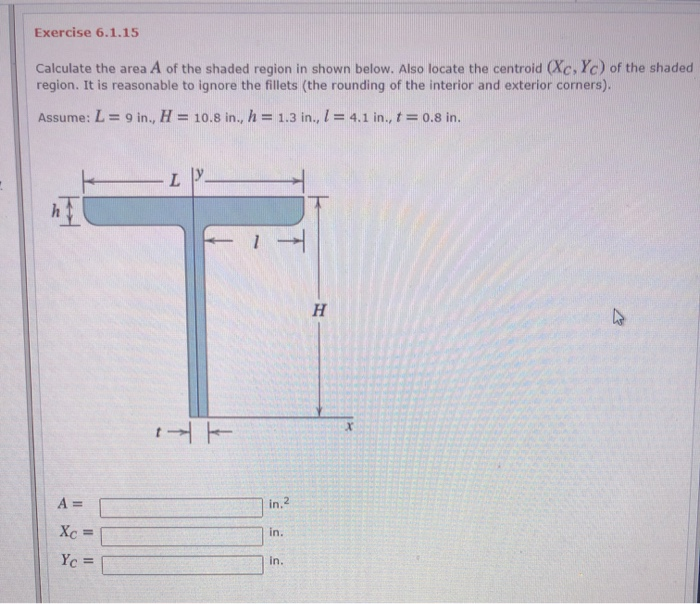Exercise 6.1.15 Calculate the area A of the shaded region in shown below. Also locate the centroid (Xc, Yc) of the shaded region. It is reasonable to ignore the fillets (the rounding of the interior and exterior corners). Assume: L = 9 in., H = 10.8 in., h = 1.3 in., 1 = 4.1 in., t = 0.8 in. L H A= in.2 Xc in. Yc = in.

• ### Calculate the area A and locate the centroid (X, Yc) of the shaded region shown below....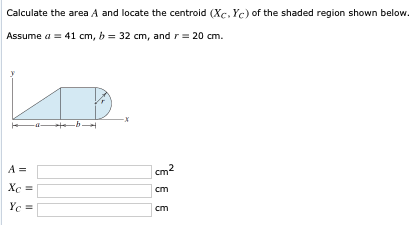Calculate the area A and locate the centroid (X, Yc) of the shaded region shown below. Assume a = 41 cm, b = 32 cm, and r = 20 cm. cm cm XC = Yc = cm

• ### Part A Locate the centroid of the shaded area. (Figure 1) Express your answer in terms...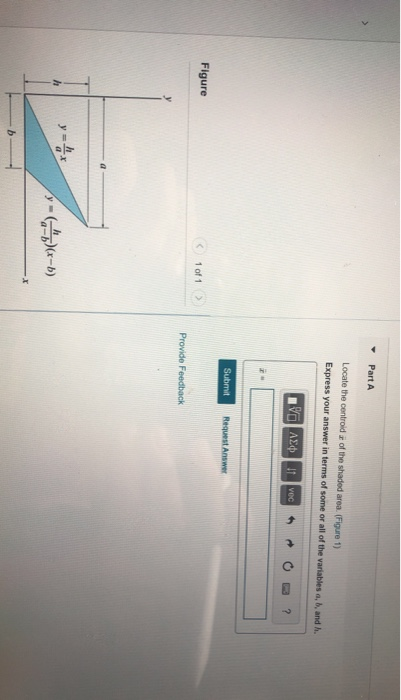Part A Locate the centroid of the shaded area. (Figure 1) Express your answer in terms of some or all of the variables a, b, and h. να ΑΣφ| Vec Submit Request Answer Figure 1 of 1> Provide Feedback a y - b) X-JAR dA JadA S-7.1de bh ai h ia t y=ha-ta'r ha- A-4a Tari .rat a-b -Σα a-i . ala-y at he a-b hla-h/ ah 241 2a-b) 24-b2la- ha 2athtikba + X- la-1) ahrbh-aht2bL 2(4-6/ hab

Free Homework App